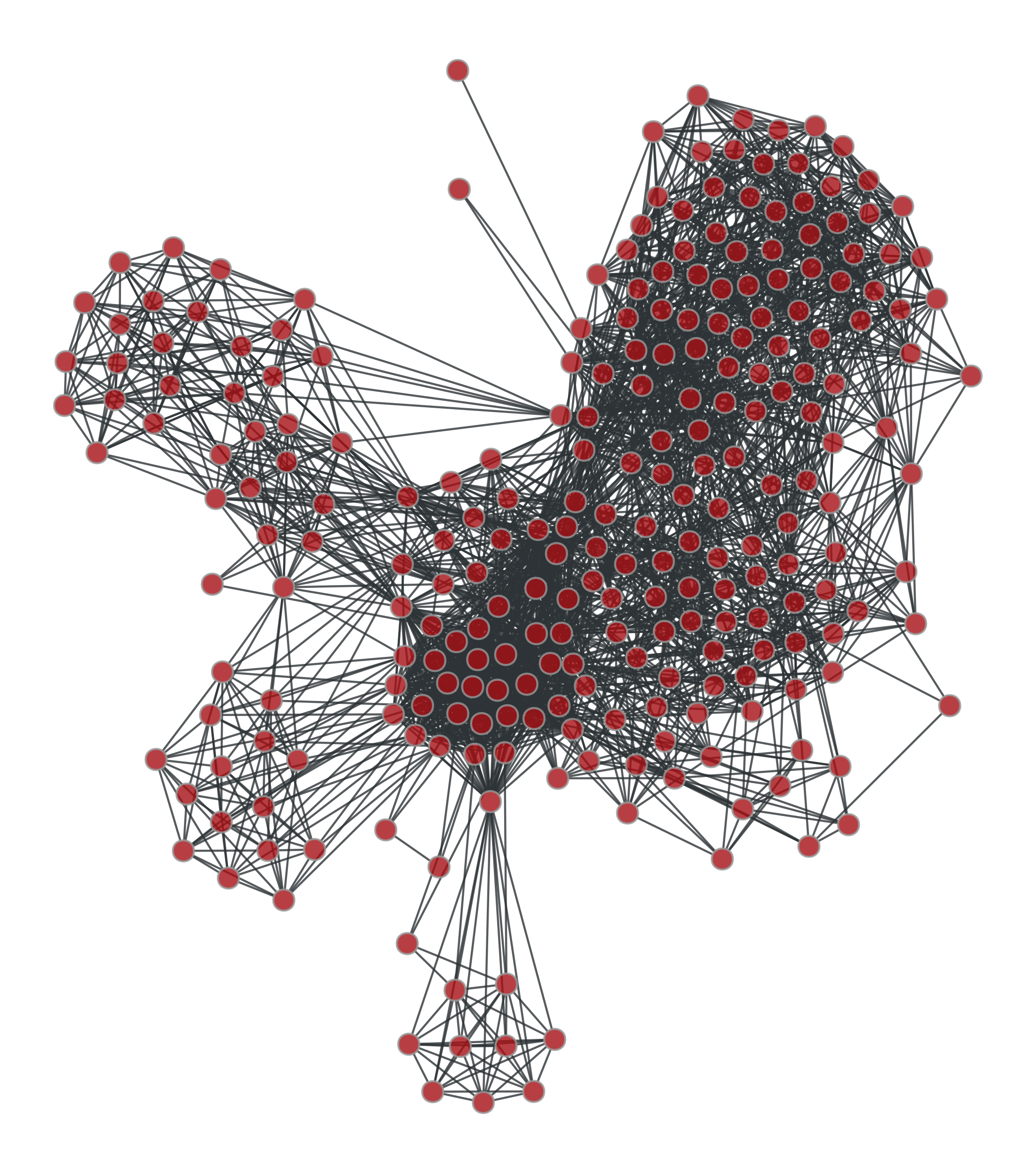# graph_tool.generation.line_graph#

graph_tool.generation.line_graph(g)[source]#

Return the line graph of the given graph g.

Notes

Given an undirected graph G, its line graph L(G) is a graph such that:

• Each vertex of L(G) represents an edge of G; and

• Two vertices of L(G) are adjacent if and only if their corresponding edges share a common endpoint (“are adjacent”) in G.

For a directed graph, the second criterion becomes:

• Two vertices representing directed edges from u to v and from w to x in G are connected by an edge from uv to wx in the line digraph when v = w.

References

Examples

```>>> g = gt.collection.data["lesmis"]
>>> lg, vmap = gt.line_graph(g)
>>> pos = gt.graph_draw(lg, output="lesmis-lg.pdf")
```Line graph of the coappearance of characters in Victor Hugo’s novel “Les Misérables”.#